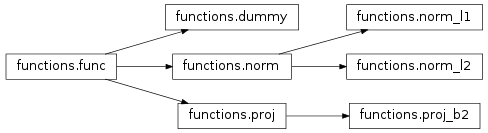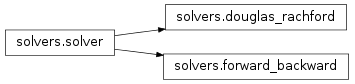# Reference guide¶

## Toolbox overview¶

PyUNLocBoX is a convex optimization toolbox using proximal splitting methods. It is a port of the Matlab UNLocBoX toolbox.

The toolbox is organized around two classes hierarchies : the functions and the solvers. Instantiated functions represent convex functions to optimize. Instantiated solvers represent solving algorithms. The pyunlocbox.solvers.solve() solving function takes as parameters a solver object and some function objects to actually solve the optimization problem.

The pyunlocbox package is divided into the following modules :

Following is a typical usage example who solves an optimization problem composed by the sum of two convex functions. The functions and solver objects are first instantiated with the desired parameters. The problem is then solved by a call to the solving function.

>>> import pyunlocbox
>>> f1 = pyunlocbox.functions.norm_l2(y=[4, 5, 6, 7])
>>> f2 = pyunlocbox.functions.dummy()
>>> solver = pyunlocbox.solvers.forward_backward()
>>> ret = pyunlocbox.solvers.solve([f1, f2], [0, 0, 0, 0], solver, atol=1e-5)
Solution found after 10 iterations :
objective function f(sol) = 7.460428e-09
last relative objective improvement : 1.624424e+03
stopping criterion : ATOL
>>> ret['sol']
array([ 3.99996922,  4.99996153,  5.99995383,  6.99994614])


## Functions module¶

This module implements function objects which are then passed to solvers. The func base class defines the interface whereas specialised classes who inherit from it implement the methods. These classes include :

• dummy: A dummy function object which returns 0 for the _eval(), _prox() and _grad() methods.
• norm: Norm operators base class.
• norm_l1: L1-norm who implements the _eval() and _prox() methods.
• norm_l2: L2-norm who implements the _eval(), _prox() and _grad() methods.
• proj: Projection operators base class.
• proj_b2: Projection on the L2-ball who implements the _eval() and _prox() methods.## Solvers module¶

This module implements solver objects who minimize an objective function. Call solve() to solve your convex optimization problem using your instantiated solver and functions objects. The solver base class defines the interface of all solver objects. The specialized solver objects inherit from it and implement the class methods. The following solvers are included :